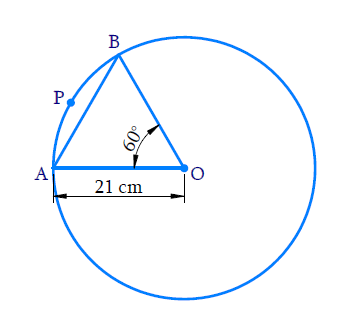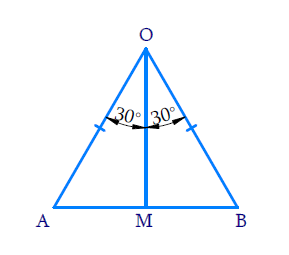# Ex.12.2 Q5 Areas Related to Circles Solution - NCERT Maths Class 10

## Question

In a circle of radius $$21\,\rm {cm,}$$ an arc subtends an angle of $$60^\circ$$ at the centre. Find:

(i) The length of the arc

(ii) Area of the sector formed by the arc

(iii) Area of the segment formed by the corresponding chord

Video Solution
Areas Related To Circles
Ex 12.2 | Question 5

## Text Solution

What is known?

Radius of the circle and angle subtended by the arc at the center.

What is unknown?

(i) Length of the arc

(ii) Area of the sector formed by the arc

(iii) Area of the segment formed by the corresponding chord

Reasoning:

In a circle with radius r and angle at the center with degree measure $$\theta$$;

(i) Length of the arc\begin{align}&= \frac{{\rm{\theta }}}{{360}^{\rm{o}}} \times 2\pi {r}\end{align}

(ii) Area of the sector\begin{align} = \frac{{\rm{\theta }}}{{360}^{\rm{o}}} \times \pi {r^2}\end{align}

(iii) Area of the segment = Area of the sector- Area of the corresponding triangle

Draw a figure to visualize the problemHere, $$\rm{}r= 21\,cm$$$$\theta = {60^\circ}$$

\begin{align} &= {\frac{\theta }{{{{360}^\circ }}} \times 2\pi r}\\&{ = \frac{{2\pi r}}{6}}\end{align}

\begin{align}&{ = \frac{\theta }{{{{360}^\circ }}} \times \pi {r^2}}\\&{ = \frac{{\pi {r^2}}}{6}}\end{align}Visually it’s clear from the figure that;

Area of the segment $${APB} =$$Area of sector $${OAPB} -\,$$Area of $$\Delta {OAB}$$Draw $${OM}\, \bot \,{AB}$$

Now consider$$\Delta {OAM}$$ and $$\Delta {OBM}$$

$$OA\,=\,OB$$(radii of a circle)

$${OM}\,= \,{OM}$$(Common)

\begin{align} &\angle {{OMA}}\!=\!\!\angle {{OMB}}\!=\!{90^ \circ }\left(\because\!{\,{{OM}} \bot {{AB}}} \!\right)\\ \end{align}

$$\therefore \,\,\Delta {{OAM}} \cong {{\Delta OBM}}$$(By RHS congruency)

\begin{align}\begin{bmatrix}{\cos {30}^\circ } = \frac{{OM}}{{OA}} \\ {\text{ or }} \\ \text{OM}= {\text{r}}\cos {{30}^\circ } = \frac{{r\sqrt 3 }}{2}\end{bmatrix}\end{align}

\begin{align}{AM} &= {r \times \frac{1}{2} = \frac{r}{2}}\end{align}

$$\text{Area of}\,\Delta OAB$$\begin{align}&= \frac{1}{2} \times AB \times OM\\&= \frac{{\sqrt 3 }}{4}{r^2}\end{align}

Steps:Radius $${(r)} = 21 \,{cm}$$ $$\theta = {60^o}$$

i) Length of the arc APB

\begin{align}&= \frac{\theta }{{{{360}^o}}} \times 2\pi r\\ &= \frac{{{{60}^\circ }}}{{{{360}^\circ }}} \times 2 \times \frac{{22}}{7} \times 21\rm{cm}\\&= 22\,{\text{cm}}\end{align}

ii) Area of the sector, AOBP

\begin{align}& = \frac{\theta }{{{{360}^o}}} \times \pi {r^2}\\ &= \frac{{{{60}^o}}}{{{{360}^o}}} \times \frac{{22}}{7} \times 21 \times 21\rm{cm^2}\\&= 231\,{\text{c}}{{\text{m}}^2}\end{align}

iii) Area of the segment = Area of the sector - area of the triangle AOB

To find area of the segment, we need to find thearea of $$\Delta {OAB}$$Draw $$OM \bot AB$$

Consider $$\Delta OAM{\text{ and }}\Delta OMB$$

$$\angle {OMA} = \angle {OMB} = {90^o}$$ (Since $${OM} \,\bot\, {AB}$$)

Therefore $$\Delta OMB \cong \Delta OMA$$(By RHS Congruency)

So, $${AM} = {MB}$$ (Corresponding parts of congruent triangles)

\begin{align}\angle OMB = \angle OMA = \frac{1}{2} \times {60^o} = {30^o}\end{align}

In $$\Delta {AOM}$$

\begin{align}{\cos {{30}^\circ } }&{= \frac{{OM}}{{OA}}{\text{ or OM}} = r\frac{{\sqrt 3 }}{2}}\\{\sin {{30}^\circ }}&\!\!=\! {\frac{{AM}}{{OA}}{\text{ or AM}}\!=\!\! \frac{1}{2}\!\times\! r\!=\!\!\frac{r}{2}}\\{ \Rightarrow \quad{\text{AB}}}&= {2{\text{AM}} = 2\frac{r}{2} = r}\end{align}

\begin{align}{\rm{AB}} &= 2{\rm{AM}}\\AB &= 2 \times \frac{1}{2}r\\AB &= r\end{align}

Therefore, Area of $$\Delta {AOB}$$

\begin{align}&= \frac{1}{2} \times AB \times OM\\& = {\frac{1}{2} \times {r} \times \frac{{r\sqrt 3 }}{2}}\\& = {\frac{{21 \times 21\sqrt 3 }}{4}}\\ &={ \frac{{441\sqrt 3 }}{4}{\text{c}}{{\text{m}}^2}}\end{align}

Area of the segment

\begin{align}= \left( {231 - \frac{{441\sqrt 3 }}{4}} \right)c{m^2}\end{align}

Learn from the best math teachers and top your exams

• Live one on one classroom and doubt clearing
• Practice worksheets in and after class for conceptual clarity
• Personalized curriculum to keep up with school